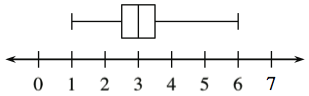### Home > INT3 > Chapter 3 > Lesson 3.1.1 > Problem3-23

3-23.

LIAR, LIAR, PANTS...

A random sample of $11$ designer men’s pants and jeans were selected from a department store. The marked size of the waist and the actual (measured) size of the waistbands were recorded (inches) and the difference in the measurements was computed.

Measured

Marked

Difference

$37$

$36$

$1$

$36$

$34$

$2$

$41$

$38$

$3$

$38.5$

$36$

$2.5$

$39.5$

$36$

$3.5$

$39$

$36$

$3$

$35.5$

$32$

$3.5$

$39$

$36$

$3$

$38.5$

$36$

$2.5$

$36$

$30$

$6$

$36$

$32$

$4$

1. Create a graphical representation of the data represented in the “difference” column.

Use the eTool below to solve the problem.
Click the link at right for the full version of the eTool: 3-23 HW eTool2. Describe the center, shape, spread, and outliers of the distribution.

See the diagram in part (a).

3. What likely conclusions can you draw from this data?

Think of why a company might adjust the sizes of their pants.

Use the eTool below to solve the problem.
Click the link at right for the full version of the eTool: 3-23 HW eTool